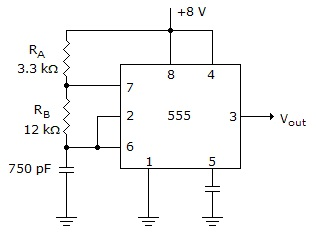# Digital Electronics - Multivibrators and 555 Timer - Discussion

### Discussion :: Multivibrators and 555 Timer - General Questions (Q.No.13)

13.

Determine tHI and tLO for the circuit given below.[A]. tHI = 7.95s, tLO = 6.24s [B]. tHI = 6.24s, tLO = 7.95s [C]. tHI = 3.97s, tLO = 3.21s [D]. tHI = 3.21s, tLO = 3.97s

Explanation:

No answer description available for this question.

 Manju said: (Sep 7, 2011) High time=ln(2)*C*(Ra+Rb) Low time=ln(2)*C*(Rb) All values given.. just subs

 Titos said: (Jul 27, 2013) High time = 0.693(Ra+Rb)*C. Low time = 0.693*Rb*C. Simply substitute with the given values.

 Ravi said: (Jul 21, 2017) Thank you @Titos.

 Adarsh said: (Jul 18, 2019) Thanks for explaining it.